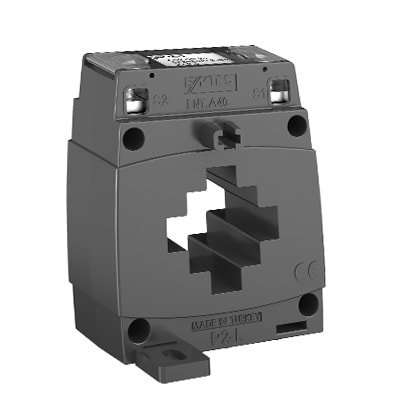# ENT.A40MN Series

 Secondary Current 5A 1A Primary Current (A) Nominal Power(VA) Class Class 3 1 0,5 3 1 0,5 100 1 X X X X ✓ X 1,5 X ✓ X X X X 2 X X X X X X 125 1,5 X ✓ X X ✓ X 2,5 X ✓ X X X X 150 1,5 X ✓ X X ✓ X 2,5 X X X X X X 200 1,5 X X ✓ X X ✓ 2,5 X X ✓ X X X 250 1,5 X X ✓ X X X 2,5 X X ✓ X X ✓ 300 2,5 X X X X X ✓ 5 X X ✓ X X 400 5 X X ✓ X X ✓ 500 5 X X ✓ X X ✓ 600 5 X X ✓ X X X
 Secondary Current 5A 1A Primary Current (A) Nominal Power(VA) Class Class 3 1 0,5 3 1 0,5 100 1 X X X X ✓ X 1,5 X ✓ X X X X 2 X X X X X X 125 1,5 X ✓ X X ✓ X 2,5 X ✓ X X X X 150 1,5 X ✓ X X ✓ X 2,5 X X X X X X 200 1,5 X X ✓ X X ✓ 2,5 X X ✓ X X X 250 1,5 X X ✓ X X X 2,5 X X ✓ X X ✓ 300 2,5 X X X X X ✓ 5 X X ✓ X X 400 5 X X ✓ X X ✓ 500 5 X X ✓ X X ✓ 600 5 X X ✓ X X X
OTHER PRODUCTS
SUPPORT AND DOCUMENTS
Get Offer
Let Us Call You